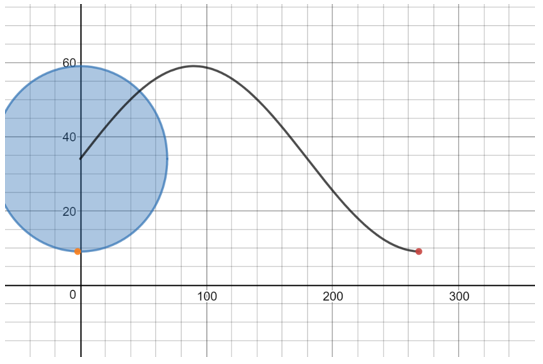Match Fishtank is now Fishtank Learning!

# Unit Circle and Trigonometric Functions

## Objective

Sketch sine graphs to model contextual situations and identify features of sine graphs.

## Common Core Standards

### Core Standards

?

• F.IF.B.4 — For a function that models a relationship between two quantities, interpret key features of graphs and tables in terms of the quantities, and sketch graphs showing key features given a verbal description of the relationship. Key features include: intercepts; intervals where the function is increasing, decreasing, positive, or negative; relative maximums and minimums; symmetries; end behavior; and periodicity. Modeling is best interpreted not as a collection of isolated topics but in relation to other standards. Making mathematical models is a Standard for Mathematical Practice, and specific modeling standards appear throughout the high school standards indicated by a star symbol (★). The star symbol sometimes appears on the heading for a group of standards; in that case, it should be understood to apply to all standards in that group.

• F.IF.C.7.E — Graph exponential and logarithmic functions, showing intercepts and end behavior, and trigonometric functions, showing period, midline, and amplitude.

• F.TF.A.4 — Use the unit circle to explain symmetry (odd and even) and periodicity of trigonometric functions.

• F.TF.B.5 — Choose trigonometric functions to model periodic phenomena with specified amplitude, frequency, and midline. Modeling is best interpreted not as a collection of isolated topics but in relation to other standards. Making mathematical models is a Standard for Mathematical Practice, and specific modeling standards appear throughout the high school standards indicated by a star symbol (★). The star symbol sometimes appears on the heading for a group of standards; in that case, it should be understood to apply to all standards in that group.

?

• G.SRT.C.6

## Criteria for Success

?

1. Identify appropriate situations for a sinusoidal model.
2. Connect sine functions with the height of a rotating object over time.
3. Identify midline, phase shift, period, and amplitude of sine functions.
4. Sketch a sine graph to model a contextual situation.
5. Identify sine functions as an example of periodic behavior.

## Tips for Teachers

?

• The basic idea of a periodic function (one that repeats) will be new to students. Start connecting the idea of the fact that it repeats with the idea that the angles around the circle repeat. (F-TF.4)
• Check out these videos: "Invention of the Ferris Wheel" on Travel Channel and "Features of Sinusoidal Functions" on Khan Academy.

## Anchor Problems

?

### Problem 1

Show the first video of Ferris Wheel by Dan Meyer.

Graph the height of the red car off of the ground over time. Where will the red car be after three minutes?

#### References

101Questions Ferris Wheel

Ferris Wheel by Dan Meyer is made available on 101Questions under the CC BY 3.0 license. Accessed Feb. 26, 2018, 10:38 a.m..

### Problem 2What features of the function do you see? What type of Ferris wheel would this correspond to?

## Problem Set

?

The following resources include problems and activities aligned to the objective of the lesson that can be used to create your own problem set.

• Include problems connecting graphs and contextual situations.
• Include problems finding periods of graphs and extrapolating to emphasize periodic behavior.
• Include problems that measure the x-axis both in time and in degrees.